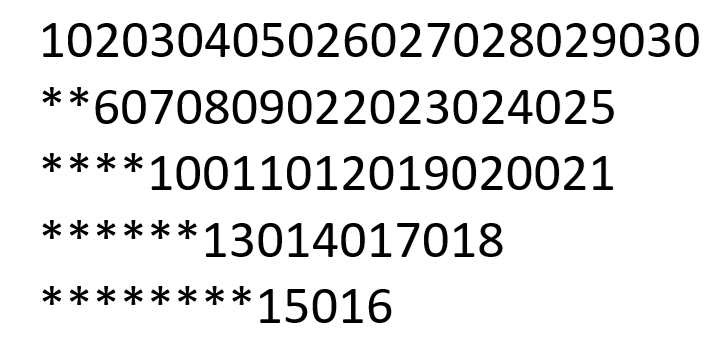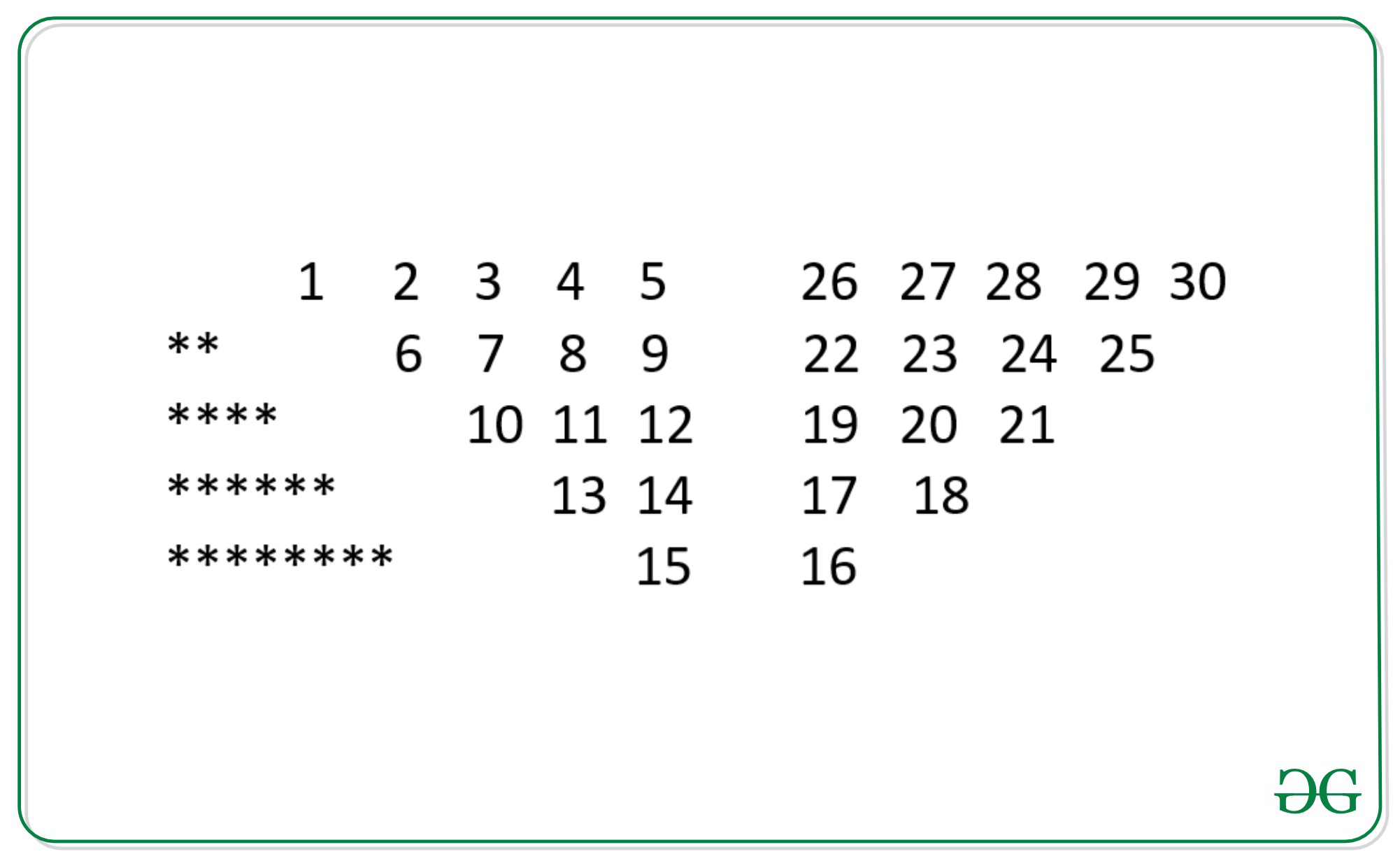# Program to print the pattern 1020304017018019020 **50607014015016 ****809012013 ******10011…

Given an integer N, the task is to print the below pattern for the given value of N.

For N = 5 Below is the given pattern:Examples:

Input: N = 4
Output:
1020304017018019020
**50607014015016
****809012013
******10011

Input: N = 3
Output:
10203010011012
**4050809
****607

Approach: The idea is to understand the logic behind the given pattern is stated below:

\By looking closely we see that by replacing in-between zeroes with spaces the pattern can be seen more clearly. The pattern is further divided into three different patterns.

1. Case 1: Asterisk (*) character pattern follows a sequence from 0 and adding two more asterisks in each row, where the row is equal to N.
2. Case 2: In this part, the pattern is very simple to understand i.e the number of columns and rows will be equal to N and follows a sequence like 1, 2, 3, 4, 5…
3. Case 3: Follow-up or bottom-up sequence is its interesting part where the numbers are represented from bottom to top.Below is the implementation of the above approach:

## C++

 `// C++ implementation to print ` `// the given pattern ` `#include ` `using` `namespace` `std; `   `// Function to find the sum of ` `// N integers from 1 to N ` `int` `sum(``int` `n) ` `{ ` `    ``return` `n * (n - 1) / 2; ` `} `   `// Function to print the given ` `// pattern ` `void` `BSpattern(``int` `N) ` `{ ` `    ``int` `Val = 0, Pthree = 0, ` `        ``cnt = 0, initial; `   `    ``string s = ``"**"``; `   `    ``// Iterate over [0, N - 1] ` `    ``for` `(``int` `i = 0; i < N; i++) { ` `        ``cnt = 0; `   `        ``// Sub-Pattern - 1 ` `        ``if` `(i > 0) { ` `            ``cout << s; ` `            ``s += ``"**"``; ` `        ``} `   `        ``// Sub-Pattern - 2 ` `        ``for` `(``int` `j = i; j < N; j++) { `   `            ``// Count the number of element ` `            ``// in rows and sub-pattern 2 and 3 ` `            ``// will have same rows ` `            ``if` `(i > 0) { ` `                ``cnt++; ` `            ``} ` `            ``// Increment Val to print the ` `            ``// series 1, 2, 3, 4, 5 ... ` `            ``cout << ++Val; ` `            ``cout << 0; ` `        ``} `   `        ``// To get the first element of sub ` `        ``// pattern 3 find the sum of first N-1 ` `        ``// elements first N-1 elements in row1 ` `        ``// previous of Sub-Pattern 2 `   `        ``// Finally, add the (N-1)th element ` `        ``// i.e., 5 and increment it by 1 ` `        ``if` `(i == 0) { ` `            ``int` `Sumbeforelast = sum(Val) * 2; ` `            ``Pthree = Val + Sumbeforelast + 1; ` `            ``initial = Pthree; ` `        ``} `   `        ``// Initial is used to give the initial ` `        ``// value of the row in Sub-Pattern 3 ` `        ``initial = initial - cnt; `   `        ``Pthree = initial; `   `        ``// Sub-Pattern 3 ` `        ``for` `(``int` `k = i; k < N; k++) { `   `            ``cout << Pthree++; `   `            ``// Skip printing zero at the last ` `            ``if` `(k != N - 1) { ` `                ``cout << 0; ` `            ``} ` `        ``} `   `        ``cout << ``"\n"``; ` `    ``} ` `} `   `// Driver Code ` `int` `main() ` `{ ` `    ``// Given N ` `    ``int` `N = 5; `   `    ``// Function Call ` `    ``BSpattern(N); ` `    ``return` `0; ` `} `

## Java

 `// Java implementation to print ` `// the given pattern ` `import` `java.util.*;`   `class` `GFG{`   `// Function to find the sum of` `// N integers from 1 to N` `static` `int` `sum(``int` `n)` `{` `    ``return` `n * (n - ``1``) / ``2``;` `}`   `// Function to print the given` `// pattern` `static` `void` `BSpattern(``int` `N)` `{` `    ``int` `Val = ``0``, Pthree = ``0``,` `        ``cnt = ``0``, initial = -``1``;`   `    ``String s = ``"**"``;`   `    ``// Iterate over [0, N - 1]` `    ``for``(``int` `i = ``0``; i < N; i++) ` `    ``{` `        ``cnt = ``0``; ` `        `  `        ``// Sub-Pattern - 1 ` `        ``if` `(i > ``0``)` `        ``{ ` `            ``System.out.print(s);` `            ``s += ``"**"``;` `        ``}`   `        ``// Sub-Pattern - 2` `        ``for``(``int` `j = i; j < N; j++)` `        ``{` `            `  `            ``// Count the number of element ` `            ``// in rows and sub-pattern 2 ` `            ``// and 3 will have same rows ` `            ``if` `(i > ``0``) ` `            ``{ ` `                ``cnt++;` `            ``}` `            `  `            ``// Increment Val to print the` `            ``// series 1, 2, 3, 4, 5 ...` `            ``System.out.print(++Val);` `            ``System.out.print(``"0"``);` `        ``}`   `        ``// To get the first element of sub` `        ``// pattern 3 find the sum of first N-1` `        ``// elements first N-1 elements in row1` `        ``// previous of Sub-Pattern 2`   `        ``// Finally, add the (N-1)th element` `        ``// i.e., 5 and increment it by 1` `        ``if` `(i == ``0``) ` `        ``{` `            ``int` `Sumbeforelast = sum(Val) * ``2``;` `            ``Pthree = Val + Sumbeforelast + ``1``;` `            ``initial = Pthree;` `        ``}`   `        ``// Initial is used to give the initial` `        ``// value of the row in Sub-Pattern 3` `        ``initial = initial - cnt;`   `        ``Pthree = initial;`   `        ``// Sub-Pattern 3` `        ``for``(``int` `k = i; k < N; k++)` `        ``{` `            ``System.out.print(Pthree++);`   `            ``// Skip printing zero at the last` `            ``if` `(k != N - ``1``) ` `            ``{` `                ``System.out.print(``"0"``);` `            ``}` `        ``}` `        ``System.out.println();` `    ``}` `}`   `// Driver code` `public` `static` `void` `main(String[] args)` `{` `    `  `    ``// Given N` `    ``int` `N = ``5``;` `    `  `    ``// Function call` `    ``BSpattern(N);` `}` `}`   `// This code is contributed by offbeat`

## Python3

 `# Python3 implementation to print ` `# the given pattern `   `# Function to find the sum of ` `# N integers from 1 to N ` `def` `sum``(n): ` `    `  `    ``return` `n ``*` `(n ``-` `1``) ``/``/` `2` ` `  `# Function to prthe given ` `# pattern ` `def` `BSpattern(N):` `    `  `    ``Val ``=` `0` `    ``Pthree ``=` `0``, ` `    ``cnt ``=` `0`  `    ``initial ``=` `-``1` `    ``s ``=` `"**"` ` `  `    ``# Iterate over [0, N - 1] ` `    ``for` `i ``in` `range``(N): ` `        ``cnt ``=` `0` ` `  `        ``# Sub-Pattern - 1 ` `        ``if` `(i > ``0``): ` `            ``print``(s, end ``=` `"")` `            ``s ``+``=` `"**"` `         `  `        ``# Sub-Pattern - 2 ` `        ``for` `j ``in` `range``(i, N): ` `            `  `            ``# Count the number of element ` `            ``# in rows and sub-pattern 2 and 3 ` `            ``# will have same rows ` `            ``if` `(i > ``0``): ` `                ``cnt ``+``=` `1` `             `  `            ``# Increment Val to prthe ` `            ``# series 1, 2, 3, 4, 5 ...` `            ``Val ``+``=` `1` `            ``print``(Val, end ``=` `"")` `            ``print``(``0``, end ``=` `"") ` `         `  `        ``# To get the first element of sub ` `        ``# pattern 3 find the sum of first N-1 ` `        ``# elements first N-1 elements in row1 ` `        ``# previous of Sub-Pattern 2 ` ` `  `        ``# Finally, add the (N-1)th element ` `        ``# i.e., 5 and increment it by 1 ` `        ``if` `(i ``=``=` `0``): ` `            ``Sumbeforelast ``=` `sum``(Val) ``*` `2` `            ``Pthree ``=` `Val ``+` `Sumbeforelast ``+` `1` `            ``initial ``=` `Pthree ` `         `  `        ``# Initial is used to give the initial ` `        ``# value of the row in Sub-Pattern 3 ` `        ``initial ``=` `initial ``-` `cnt ` ` `  `        ``Pthree ``=` `initial ` ` `  `        ``# Sub-Pattern 3 ` `        ``for` `k ``in` `range``(i, N): ` `            ``print``(Pthree, end ``=` `"")` `            ``Pthree ``+``=` `1` ` `  `            ``# Skip printing zero at the last ` `            ``if` `(k !``=` `N ``-` `1``): ` `                ``print``(``0``, end ``=` `"")` `                `  `        ``print``() ` `        `  `# Driver Code `   `# Given N ` `N ``=` `5` ` `  `# Function call ` `BSpattern(N)`   `# This code is contributed by sanjoy_62`

## C#

 `// C# implementation to print ` `// the given pattern ` `using` `System;` `class` `GFG{` ` `  `// Function to find the sum of` `// N integers from 1 to N` `static` `int` `sum(``int` `n)` `{` `    ``return` `n * (n - 1) / 2;` `}` ` `  `// Function to print the given` `// pattern` `static` `void` `BSpattern(``int` `N)` `{` `    ``int` `Val = 0, Pthree = 0,` `        ``cnt = 0, initial = -1;` ` `  `    ``String s = ``"**"``;` ` `  `    ``// Iterate over [0, N - 1]` `    ``for``(``int` `i = 0; i < N; i++) ` `    ``{` `        ``cnt = 0; ` `         `  `        ``// Sub-Pattern - 1 ` `        ``if` `(i > 0)` `        ``{ ` `            ``Console.Write(s);` `            ``s += ``"**"``;` `        ``}` ` `  `        ``// Sub-Pattern - 2` `        ``for``(``int` `j = i; j < N; j++)` `        ``{` `             `  `            ``// Count the number of element ` `            ``// in rows and sub-pattern 2 ` `            ``// and 3 will have same rows ` `            ``if` `(i > 0) ` `            ``{ ` `                ``cnt++;` `            ``}` `             `  `            ``// Increment Val to print the` `            ``// series 1, 2, 3, 4, 5 ...` `            ``Console.Write(++Val);` `            ``Console.Write(``"0"``);` `        ``}` ` `  `        ``// To get the first element of sub` `        ``// pattern 3 find the sum of first N-1` `        ``// elements first N-1 elements in row1` `        ``// previous of Sub-Pattern 2` ` `  `        ``// Finally, add the (N-1)th element` `        ``// i.e., 5 and increment it by 1` `        ``if` `(i == 0) ` `        ``{` `            ``int` `Sumbeforelast = sum(Val) * 2;` `            ``Pthree = Val + Sumbeforelast + 1;` `            ``initial = Pthree;` `        ``}` ` `  `        ``// Initial is used to give the initial` `        ``// value of the row in Sub-Pattern 3` `        ``initial = initial - cnt;` ` `  `        ``Pthree = initial;` ` `  `        ``// Sub-Pattern 3` `        ``for``(``int` `k = i; k < N; k++)` `        ``{` `            ``Console.Write(Pthree++);` ` `  `            ``// Skip printing zero at the last` `            ``if` `(k != N - 1) ` `            ``{` `                ``Console.Write(``"0"``);` `            ``}` `        ``}` `        ``Console.WriteLine();` `    ``}` `}` ` `  `// Driver code` `public` `static` `void` `Main(String[] args)` `{` `     `  `    ``// Given N` `    ``int` `N = 5;` `     `  `    ``// Function call` `    ``BSpattern(N);` `}` `}` ` `  `// This code is contributed by shikhasingrajput`

Output:

```102030405026027028029030
**6070809022023024025
****10011012019020021
******13014017018
********15016

```

Time Complexity: O(N2)
Auxiliary Space: O(1)

Attention reader! Don’t stop learning now. Get hold of all the important DSA concepts with the DSA Self Paced Course at a student-friendly price and become industry ready.

My Personal Notes arrow_drop_upCheck out this Author's contributed articles.

If you like GeeksforGeeks and would like to contribute, you can also write an article using contribute.geeksforgeeks.org or mail your article to contribute@geeksforgeeks.org. See your article appearing on the GeeksforGeeks main page and help other Geeks.

Please Improve this article if you find anything incorrect by clicking on the "Improve Article" button below.